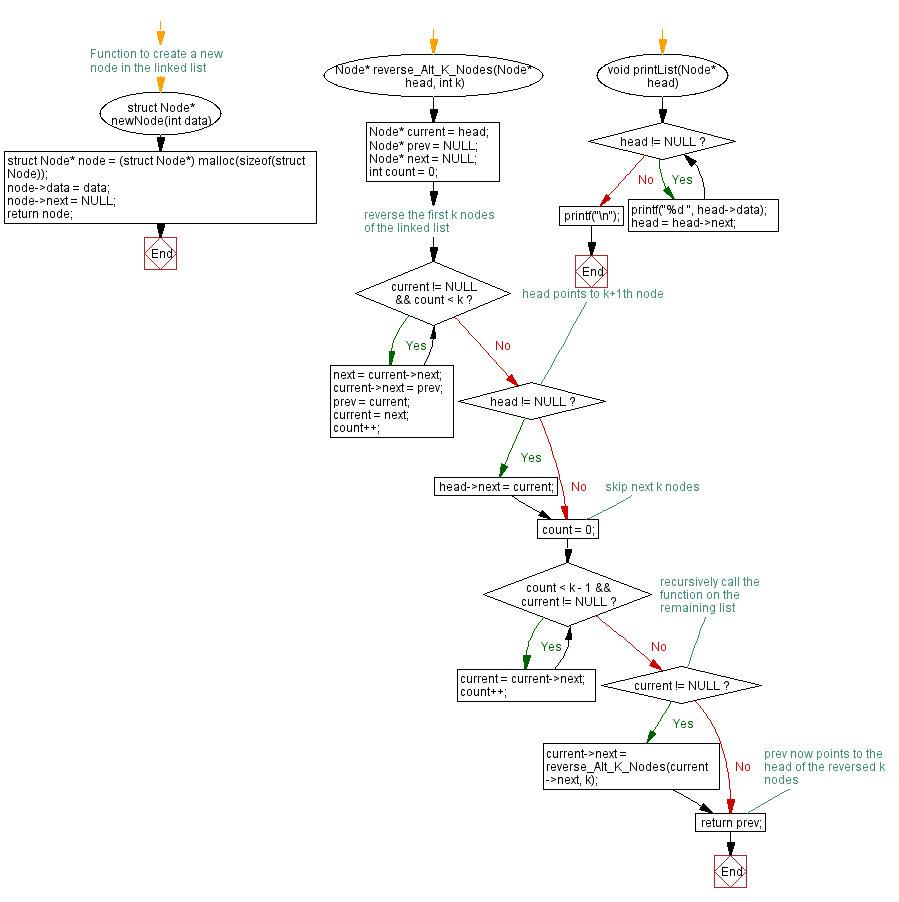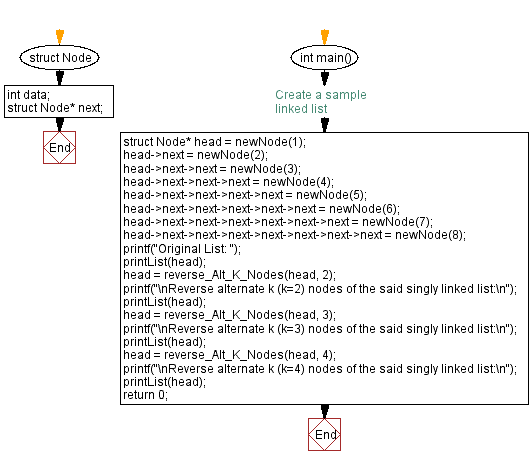﻿ C Program: Reverse alternate k nodes of a singly linked list

# C Exercises: Reverse alternate k nodes of a singly linked list

## C Singly Linked List : Exercise-41 with Solution

Write a C program to reverse alternate k nodes of a given singly linked list.

Sample Solution:

C Code:

``````#include<stdio.h>
#include <stdlib.h>

struct Node {
int data;
struct Node* next;
};

// Function to create a new node in the linked list
struct Node* newNode(int data) {
struct Node* node = (struct Node*) malloc(sizeof(struct Node));
node->data = data;
node->next = NULL;
return node;
}

Node* reverse_Alt_K_Nodes(Node* head, int k) {
Node* prev = NULL;
Node* next = NULL;
int count = 0;

/* reverse the first k nodes of the linked list */
while (current != NULL && count < k) {
next = current->next;
current->next = prev;
prev = current;
current = next;
count++;
}

/* head points to k+1th node */
}

/* skip next k nodes */
count = 0;
while (count < k - 1 && current != NULL) {
current = current->next;
count++;
}

/* recursively call the function on the remaining list */
if (current != NULL) {
current->next = reverse_Alt_K_Nodes(current->next, k);
}

/* prev now points to the head of the reversed k nodes */
return prev;
}

}
printf("\n");
}

int main() {
// Create a sample linked list

printf("Original List: ");

printf("\nReverse alternate k (k=2) nodes of the said singly linked list:\n");
printf("\nReverse alternate k (k=3) nodes of the said singly linked list:\n");
printf("\nReverse alternate k (k=4) nodes of the said singly linked list:\n");
return 0;
}
```
```

Sample Output:

```Original List: 1 2 3 4 5 6 7 8

Reverse alternate k (k=2) nodes of the said singly linked list:
2 1 3 4 6 5 7 8

Reverse alternate k (k=3) nodes of the said singly linked list:
3 1 2 4 6 5 8 7

Reverse alternate k (k=4) nodes of the said singly linked list:
4 2 1 3 6 5 8 7
```

Flowchart :C Programming Code Editor:

What is the difficulty level of this exercise?

﻿